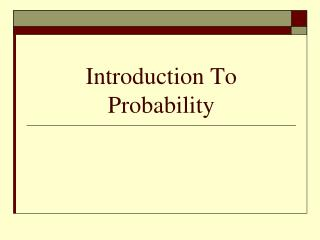DownloadDownload PresentationIntroduction To Probability

Introduction To Probability

Download PresentationIntroduction To Probability

- - - - - - - - - - - - - - - - - - - - - - - - - - - E N D - - - - - - - - - - - - - - - - - - - - - - - - - - -
Presentation Transcript

1. Introduction To Probability

2. Probability Warm-Up Homework • Venn 1 – Reading Venn diagrams • Probability Revision Try these so that I can see where you are up to • Venn 2 – Shading Venn diagrams • Venn 3 – Probabilities and set notation Year 11 Probability Pre-Test 2

3. Introductory Notes Notes: Instead of copying notes from the board a set of notes have been prepared for you. Remember to stick the example sheet into your workbook.

4. Terminology

5. The Probability Formulae Informally we work out probabilities in our head using The formal probability formula is

6. Single event probabilities Single events are situations where only one thing happens. e.g. What Pr(odd) when rolling a die?

7. Single Probability Class Exercise (Answer on Worksheet) 1. If a drawer has 10 black socks, 6 grey socks and 4 white socks event A = drawing a black sock and event B = drawing a white sock (a) What is n() (b) What is Pr(A) (c) What is n(B) (d) What is Pr (not B) (e) What is Pr(A or B) 2. The letters of the word TREACLEare each written on a piece of paper and then randomly drawn from a box. If we let event A = drawing a vowel and event B = drawing a T (a) What is n() (b) What is n(A) (c) What is Pr(B) (d) What is Pr (not B) (e) What is Pr(A or B) 20 Interesting Question What is the Sample Space? Interesting Question How can you simplify on your calculator? 4 7 3

8. Simple Probability Class Exercise • How many cards are there in a pack? … 52 • What are the suits? … hearts, diamonds, clubs & spades • What are the colours are each suit? … red (H & D) black (C & S) • How many cards in each suit? … 13 • What are the cards in each suit? … A,2,3,4,5,6,7,8,9,10,J,Q,K • How many Aces are there? … 4 • What are the picture cards? … Jack, Queen, King • How many picture cards are there? …12 • Simplify 52  4 =13 52  13 =4

9. Simple Probability Class Exercise 12. If a card is randomly drawn from a pack of cards and we let event A = drawing a jack and event B = drawing a red card (a) Draw a Venn Diagram (b) What is n() (c) What is Pr(A) (d) What is n(B) (e) What is Pr (A and B) (f) What is Pr(A or B) See the board 52 26

10. Set Notation

11. Probabilities & Set Notation Exercise If a card is randomly drawn from a pack of cards and we let event A = drawing an Ace, event B = drawing a spade, event C = drawing a picture card (a)Draw a Venn Diagram (b) What is Pr(A) (c) What is Pr(B) (d) What is Pr (A  B) (e) What is Pr(A  C) (f) What is Pr(A  C) (g) What is Pr(B C) See the board 0

12. Homework HW Probabilities and set notation